Up: ALIAS-Maple Previous: Examples

• La page de J-P. Merlet
• La page Présentation de HEPHAISTOS
• La page "Présentation" de l'INRIA

Subsections

# Compilation problems

In some cases for complex expressions the code produced by ALIAS-Maple cannot be compiled for lack of memory. C++ seems to be indeed quite sensitive to the size of the expressions.

A first possibility is to turn off the optimization flag that is used for the compilation by setting the flag `ALIAS/optimized` to 0.

If the compilation cannot still be completed you are confronted to a difficult problem: basically the only solution is to try to simplify the generated expression using auxiliary variables for terms that appear at least twice in the code. The mechanisms described in sections 2.1.2,2.3.2 may allow to perform part of this task automatically.

But many time it will be necessary to modify the C++ program that has been produced especially the procedure involving the expressions, their jacobian and Hessian. The name of this procedure are usually F, J and H respectively and are located usually in the files _F_.c, _J_.c, _H_.c.

Another possibility is to compile separately these files which are included in the main program (usually named _G.._.C). For that you will need to modify the code and makefile (which is usually named _makefile). For example for the _F_.c you will first rename this program _F_.C, add the following header:

```
#include <fstream>
#include "Functions.h"
#include "Vector.h"
#include "IntervalVector.h"
#include "IntervalMatrix.h"
#include "IntervalMatrix.h"
#include "IntegerVector.h"
#include "IntegerMatrix.h"
```
Then the makefile will be modified so that _F_.o will appear in the dependency. Hence the line:
```
_GS_:_GS_.C \$(LIB_SOLVE)
```
will be changed to:
```
_GS_:_GS_.C _F_.o \$(LIB_SOLVE)
```
Then indicate that _F_.o should be linked by adding this file name before the library flag LIB_SOLVE. Hence:
```
\$(CC) -I\$(INCL) -I\$(INCLI) _GS_.C -o _GS_ \
\$(LIB_SOLVE) -L\$(LIB) \$(LD) -lm
```
will be changed to:
```
\$(CC) -I\$(INCL) -I\$(INCLI) _GS_.C -o _GS_ \
_F_.o \$(LIB_SOLVE) -L\$(LIB) \$(LD) -lm
```

An alternative is to avoid generating C++ file for the interval evaluation of expression but rather use the ALIAS-C++ parser to get the interval evaluation of the expressions by an interpretation mechanism. This is possible for most of the ALIAS-Maple procedures by setting the variable `ALIAS/use_parser` to 1. To determine if a procedure offers this possibility check the on-line help and look if the variable `ALIAS/use_parser` is in the list of the global variables for the procedure.

# Execution problems

To run any program generated by ALIAS-Maple it is necessary that your PATH variable always include the current directory.

## Wrong results

If ALIAS-Maple returns a wrong result the most probable reason is that the theoretical system of expression has not been correctly translated into a C++ interval equivalent. This may occur if the expression involves numerical values and the value of the Maple Digits variable has a low value (by default the value is 10). With a too low value there may be a round-off of any numerical values that may indeed cause a solving problem. Hence Digits and the `ALIAS/digits` of ALIAS-Maple should be set to a large value (typically 40).

You must also be careful with expression involving rational numbers that may be not translated correctly in C++ (for example 3/9 is usually quite different from 3./9.).

## Running out of memory

Even for very large problem it is highly improbable that a program generated by ALIAS-Maple will run out of memory as soon as the single bisection mode is chosen (by setting `ALIAS/single_bisection` to a value larger than 0) and by choosing the reverse storage mode (by assigning `ALIAS/storage_mode` to a value larger than the number of variables.

## Large computation time

### Changing the formulation of the problem

Interval analysis is a very specific domain and require some expertise to be efficient. We give in this section some tricks that may help you.

As a naive user you may want to solve a specific problem but the problem that you have submitted to ALIAS may be already a transformed version of the problem you want really to solve and this transformation may be not favorable to the use of interval analysis. Focusing on the problem you want to solve is important for the use of interval analysis.

Rule: focus on the problem you want to solve

Let us give you an example: you have a third order polynomial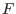whose coefficients depend on a set of parameters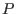and you want to find if there are values of these parameters such that the root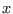of the polynomial verifies some properties. A natural way of doing that is to use the available generic analytical form of the root and check if you may find parameters such thatsatisfy the properties. In terms of interval analysis you have already transformed your problem and this may not be a good idea (the analytical form of the roots of a 3rd order polynomial is badly conditioned).

Rule: it is usually a bad idea to try to adapt to interval analysis the last step of a solving problem that has already been transformed to fit another method

Thinking backward what you want really is to verify if thesatisfying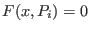satisfy the properties. Hence you should use as unknown not only thebut also the: you add an unknown but the calculation will then involve only the evaluation of the polynomial which is much more simple than the evaluation of the analytical form of the roots. Note that ALIAS C++ provides procedures to determine bounds for the unknown.

Rule: introducing new variables for simplifying expressions may be a good strategy

See section 11.2.2 for another example on how focusing on the problem may help to solve it efficiently. A good strategy is also to learn what are the main problems that have been addressed in interval analysis so that you can later on transform your problem into another one for which solving algorithms are available.

Rule: investigate which main problems have been addressed in interval analysis so that you may later on transform your problem into another one for which solving algorithms are available

Typical of such approach is to determine if a parametric matrix may include singular matrices. You may be tempted to derive the determinant of the interval matrix and then to determine if there are values of the unknowns that cancel it. But if you have not been able to manually determine these values this probably means that the determinant is quite complicated, and therefore not appropriate for interval analysis. But determining if a parametric matrix may include a singular matrix is a well-known problem in interval analysis and for solving this problem there are efficient methods that do not require the calculation of the determinant (see section 3.3.3.2).

Another case of failure occurs when the system is badly conditioned. This may occur, for example, when you have large coefficients in an equation while the values of the variable are very small: as ALIAS takes into account the round-off errors you will get large interval evaluation even for a small width of the intervals in the input interval. There is no standard way to solve the problem. The first thing you may try is to normalize the unknowns in order to reduce the size of the coefficients. A second approach may be to switch the solving problem to an optimization problem.

Another trick for system solving is to combine the initial equations to get new one. For example consider the system:

```
cons1 := a1 + a2 + a3 + 1 ;
cons2 := a1 + 2*a2*x1 + 3*a3*x1^2 + 4*x1^3 ;
cons3 := a1 + 2*a2*x2 + 3*a3*x2^2 + 4*x2^3 ;
cons4 := a1 + 2*a2*x3 + 3*a3*x3^2 + 4*x3^3 ;
cons5 := a1*(x1+x2) + a2*(x1^2 + x2^2) + a3*(x1^3 + x2^3) + x1^4 + x2^4 ;
cons6 := a1*(x2+x3) + a2*(x2^2 + x3^2) + a3*(x2^3 + x3^3) + x2^4 + x3^4 ;
cons7 := x1 + delta -x2<= 0;
cons8 := x2 + delta -x3<= 0;
```
(which is the fredtest example of our Web tests page). As it this system is difficult to solve (the computation time is over 10 minutes with a 3B increment of 0.001). Now we may notice that monomials involving a1 appear in many equations. Hence we consider adding as equations cons2-cons1, cons2-cons3 and so on. With this additional equations the computation time reduces to about 1mn 20 seconds.

Rule:Very often introducing additional constraints derived from the set of initial ones will reduce the solving time

Interval arithmetics plays evidently a large role in the computation time. The interval evaluation of mathematical operator such as sine, cosine,...is relatively expensive. If similar complex expressions appear more than once in an expression it is usually very efficient to compute it only once and to substitute it in the other places (see section 2.1.2 for a procedure that allows this manipulation).

Rule:try to avoid multiple interval evaluation of the same complex expressions

### Choosing the right heuristics

This is a very complex issue: in interval analysis we use a lot of heuristics but it is quite difficult to determine what is the right combination that will be the most efficient to solve a problem. And choosing the right parameters for these heuristics may have a very large influence on the computation time (and we mean a really large with a decrease factor of the computation time that may be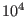).

Usually it is good policy to use filters that may be generated with simplification procedures (see section 4.2). For example the 2B-consistency, see the Hull_Consistency procedure, section 4.2.1) with a repeat factor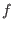that is about the diameter of the initial range divided by 1000. Similarly it is good policy to use the 3B method (see section 4.5) with an `ALIAS/Delta3B` value similar to thefactor. You may also consider looking at the ALIAS-C++ library that provide specific filters (not all of them are incorporated in ALIAS-Maple) that you may use to write your own specific simplification procedure.

## Crash

You have found an ALIAS bug. First check the error message if any. If you get a message of type Abort: Division by Zero (which originates from Bias/Profil) this means that you have tried to evaluate with interval arithmetic an expression that is not allowed: see sections 2.1.4 and 2.1.5 to correct such behavior. A call to the procedures Verify_Problem_Expression, Verify_Problem_ExpressionJ before the filtering and solving procedures will usually be sufficient to solve the problem.

In all other cases we will be happy to have a look at your program and see what has gone wrong.

The bug report should contain as much information as possible so that we can repeat your problem on our computers. Clearly this report should indicate what type of computer you are using, the operating system and the C++/Maple files that have been used. It may also be interesting to give some background information on the problem you want to solve. By giving background information on the problem we may also be able to suggest another, maybe simpler, way to solve it.

# Setting the debug option

If you cannot succeed in finding the right parameters you may use the debug option and to try to understand what is going on.

The algorithms of ALIAS are in general giving some information when running. There is a debug tool with three different levels: the first one (which is the default) is user-oriented and will help you to figure out what is going on, the second one is for expert and the third one is no debug at all. To set the debug option add to your program one of the following sentence:

```
`ALIAS/debug`:=1:
```
The value 0 indicates no debug while level 2 is the highest (1 being the default). Then, when you run your program ALIAS will print lines looking like the following one:
```
Current box (11/23,remain:13), Sol: 0 (W=0.1,73.275)
```
This indicates that the algorithm is dealing with box 11 in a list that has 23 elements. Thus 13 boxes are still to be considered as the algorithm will end when all the elements in the list have been considered.

The following part Sol:0 indicates that up to now ALIAS has found 0 solutions (for an optimization problem the value you get here is the current minima or maxima).

The last element, (W=0.1,73.275) indicates that for the current box the minimal width of the intervals in the set is 0.1 while the largest width is 73.275. With these information you may figure out what is going on in the algorithm.

Setting the debug variable to 2 will give you a large amount of information but it is reserved for experts.

the index keywords in typeset font indicate variable that are used either in the C++ library (with the exception of the C++ procedure of BIAS/Profil that are displayed in normal font) or in the Maple library. In the later case if the keyword is, for example, permute the name of the Maple variable is `ALIAS/permute`.Up: ALIAS-Maple Previous: Examples